Tutorials For Excel everyday with EVBA.info part 11[Free PDF ebook] - EBOOK VBA EXCELEVBA.info ( Ebook VBA Excel ) Welcome EVBA.info - Nothing Is Unable ... About Excel Tricks, Learning VBA Programming, Dedicated Software, Free Courses, Living Skills ...

## EVBA’s Tutorials For Excel: Rounding Times of Day by the Half Hour

Tom’s Tutorials For Excel: Rounding Times of Day by the Half Hour
Here are three pictures to show how a time of day can be rounded…
• To its nearest half-hour.
• Up, to its next half-hour.
• Down, to its last half-hour.

Picture #1 – Rounding a time to its nearest half-hour.
The formulas in range E3:F20 round their respective times in range B3:C20.
The formula in cell E3, which is copied across and down to cell F20 is
`=ROUND(B3*48,0)/48`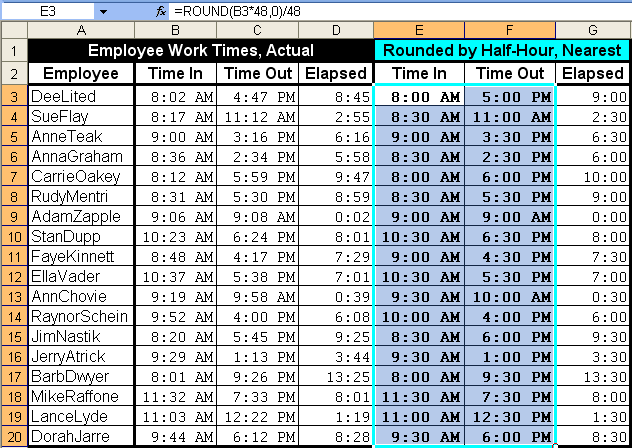Picture #2 – Rounding a time up to its next half-hour.
The formulas in range E3:F20 round their respective times in range B3:C20.
The formula in cell E3, which is copied across and down to cell F20 is
`=CEILING(B3,0.5/24)`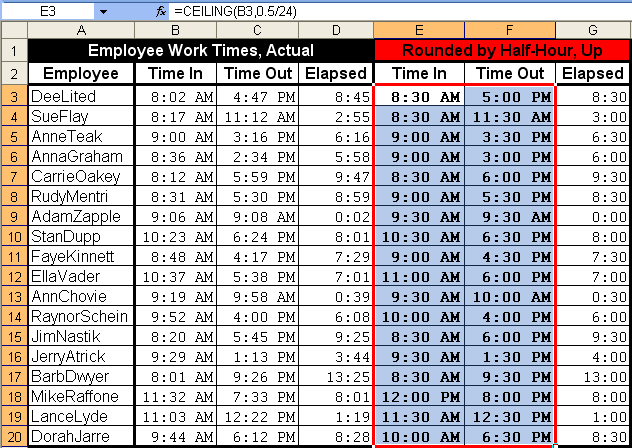Picture #3 – Rounding a time down to its last half-hour.
The formulas in range E3:F20 round their respective times in range B3:C20.
The formula in cell E3, which is copied across and down to cell F20 is
`=FLOOR(B3,0.5/24)`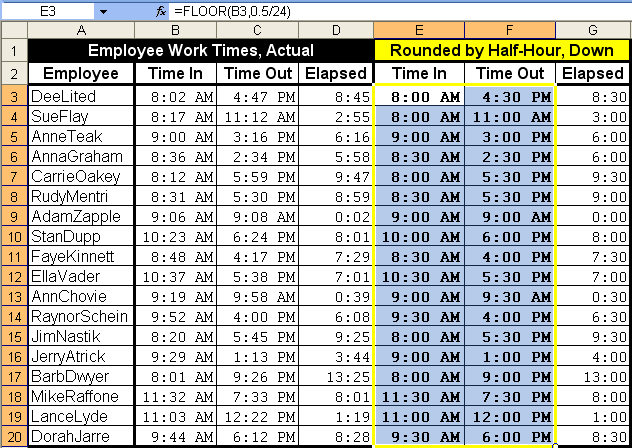Tom’s Tutorials For Excel: Rounding Elapsed Time by the Hour
Here are 3 formulas that round an elapsed time by the hour, in terms of nearest hour, up to the next hour, and down to the last (lower) hour.
In the picture, notice the differences for each employee’s elapsed time in decimalized hours, depending on if, and how, you decide to round or not round. The number 24 in the formulas relates to the count of hours that comprise a full calendar day.
The subtraction formula that rounds to the nearest hour, entered in cell E3 and copied down:
`=ROUND((C3-B3)*24,0)`
The subtraction formula that rounds up to the next hour, entered in cell F3 and copied down:
`=CEILING((C3-B3)/(1/24),1)`
Tom’s Tutorials For Excel: Rounding all Cells in a Summed Range
When you want to sum or average a range of cells that need to each be rounded beforehand, here’s an efficient alternative to rounding each cell individally. In the pictured example, daily rainfall amounts with one decimal are to be summed, based on original amounts with two decimals.
The picture shows two methods that arrive at the same result. One method uses a helper column (column C) to round each of the 31 numbers, and then a `SUM` function in cell C34 sums the helper column’s `ROUND` function formulas.
The other method uses a single array formula in cell E2 to do the entire cell-by-cell rounding process, and then sums those 31 rounded elements with no helper cells needed.
The array formula in cell E2 is `=SUM(ROUND(B3:B33,1))`.
Recall, an array formula is applied to the cell by pressing `Ctrl+Shift+Enter`, not just `Enter`.
Tom’s Tutorials For Excel: Separating Date and Time From NOW.
Here’s how you can store the date in one cell, and the time in another cell, of a source cell that contains both a date and time, such as if that source cell were holding the `NOW` function.
This is different than just copying the `NOW` function into 2 other cells and formatting those 2 other cells as date and time respectively. Formatting a cell only changes the appearance of its contents, but the underlying value in that scenario would still be both a date and time in all 3 cells.
Here, you are separating out the actual date value, and the actual time value, with this result:
Here are the steps by example.
Step 2
Maybe you’ll want your `NOW` function to constantly update itself. But just for fun, if you want to make it constant (unchanging), select the cell, press `F2`, then press `F9`, then press `Enter`.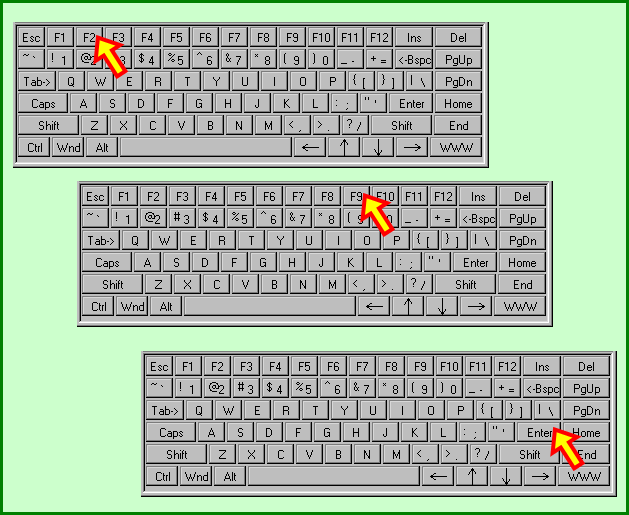Step 3
• Select the cell (B2 in this example) that will hold the date.
• In that cell, enter the formula `=INT(A2)`.
• With that cell selected, right click it and select Format Cells.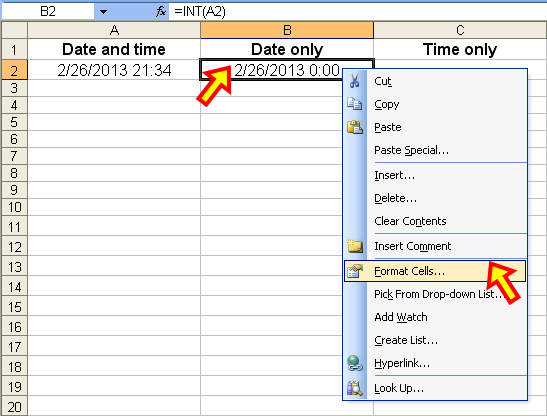Step 4
In the Format Cells dialog…
• Click onto the Number tab.
• In the Category pane, select Date.
• In the Type list, select your desired Date format.
• Click OK.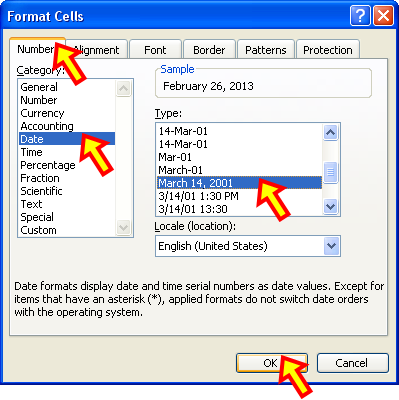Step 5
• Select the cell (C2 in this example) that will hold the time.
• In that cell, enter the formula `=MOD(A2,1)`.
• With that cell selected, right click it and select Format Cells.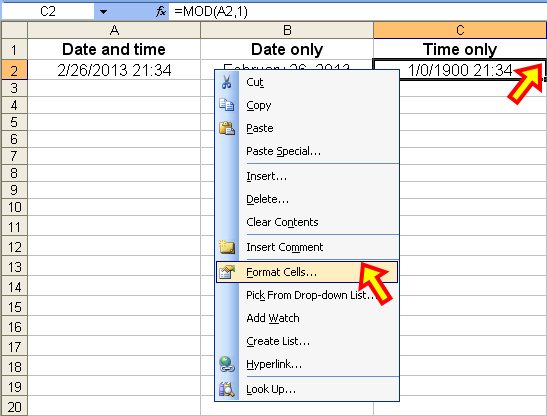Step 6
In the Format Cells dialog…
• Click onto the Number tab.
• In the Category pane, select Time.
• In the Type list, select your desired Time format.
• Click OK.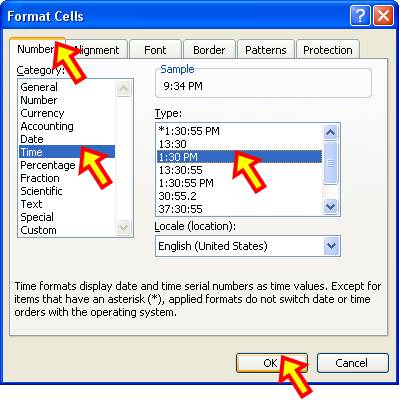Tom’s Tutorials For Excel: Sorting Email Addresses by Domain and Recipient
Yesterday, I posted this method for parsing the recipient and domain names from an email address.
As an example of why you would want to separate a recipient name from its domain name, a common marketing task is to sort the company’s list of client email targets by client name (domain) and then by recipient name of that client.
The following pair of Before and After pictures shows an unorganized random list of client email addresses, and then that same list sorted in ascending order by domain name and recipient name.
Step 3
For whichever version of Excel you are using, indicate in the Sort dialog box that you want to sort the range by Domain in ascending order as the primary field, and then by Recipient in ascending order as the secondary field. Also indicate that your selected range has a header row.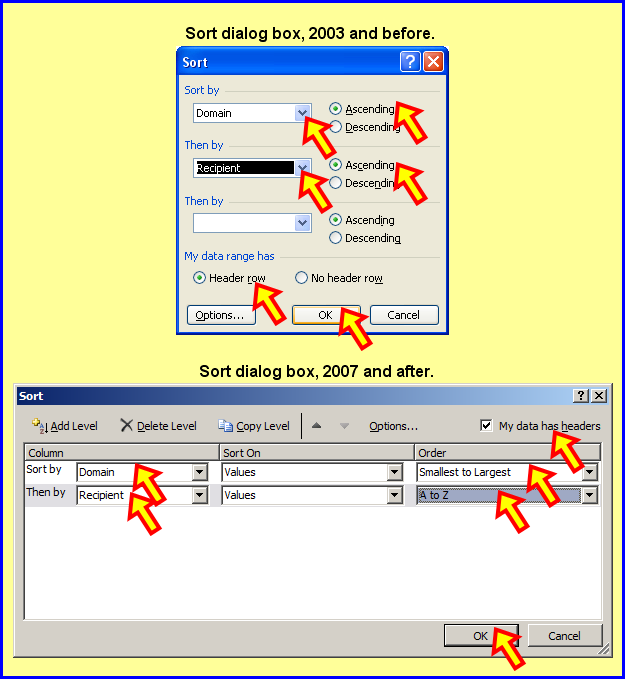Step 4
Not shown in a picture because it is optional, to delete the two helper columns.
Tom’s Tutorials For Excel: Parsing an Email Address for Recipient and Domain Names
If you have a list of email addresses and you want to parse the recipient and domain names, you can do so with the following formulas as shown in the picture. Note that the formulas will take into account the varying lengths of recipient and domain names.
With the list of email addresses in column A starting in cell A2, this formula in cell B2 and copied down as needed will return the recipient name:
`=LEFT(A2,FIND("@",A2)-1)`
This formula in cell C2 and copied down as needed will return the domain name:
`=SUBSTITUTE(A2,LEFT(A2,FIND("@",A2)),"")`
Tom’s Tutorials For Excel: Entering a Static Random Number
I previously posted these examples of data entry using random numbers.
In each of those examples, by design, the random numbers returned by the `RAND` function were volatile. That is, any change to worksheet data would recalculate those formulas, which is what you would have wanted to happen for those examples.
In some project designs, you need to enter a static random number that remains unchanged until and unless you want to change it. For that, an approach other than the `RAND` function is needed.
In the next picture, the numbers in cells A1, A2, and A3 were each produced in a static random fashion. The next three sets of steps show how this was accomplished.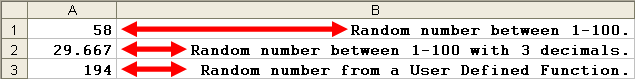Example 1 — the number in cell A1.
The quickest and easiest way to enter a static random number is to use the Immediate window.
From your keyboard, press `Alt+F11` then press `Ctrl+G`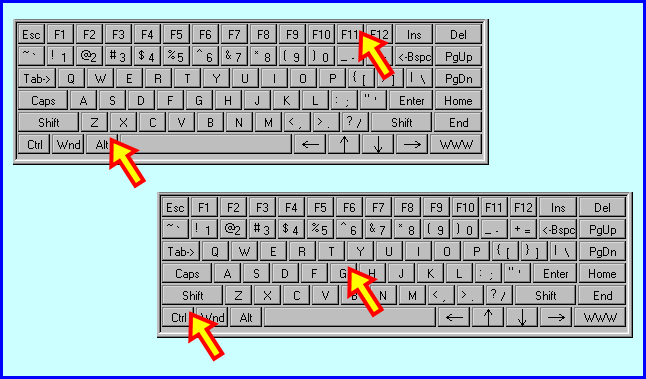I typed this line of code into the Immediate window…
`Range("A1").Value = Format(Rnd() * 99 + 1, "000")`
…to produce a static random number between 1 and 100 in cell A1.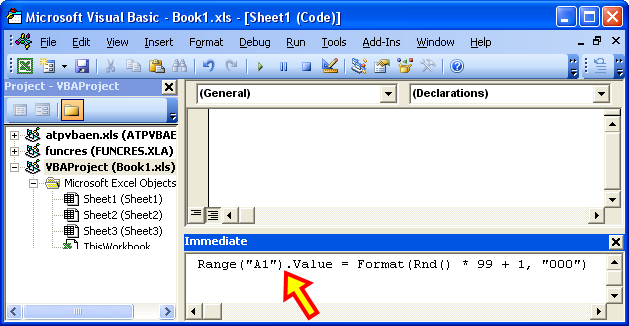To actually place that number in cell A1, hit the `Enter` key.
To return to the worksheet thereafter, press `Alt+Q`.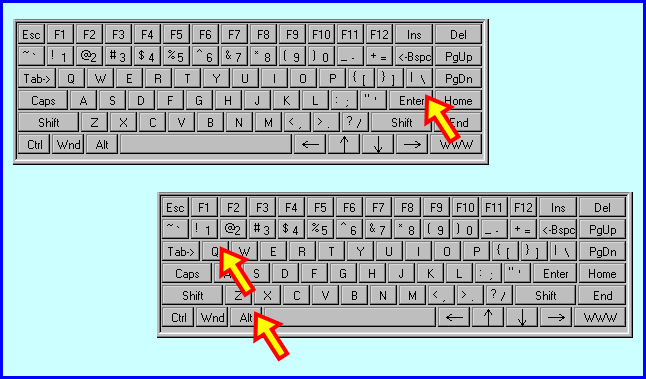Final result for Example 1: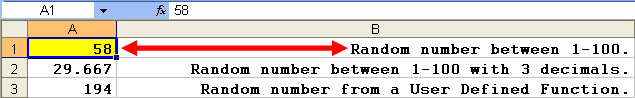Example 2 — the number in cell A2.
Following the same steps as in Example 1, from your keyboard, press `Alt+F11` then press `Ctrl+G` to access the Immediate window. Cell A2 also holds a static random number between 1 and 100, but with three decimal places using this statement:
`Range("A2").Value = Format(Rnd() * 99 + 1, "0.000")`.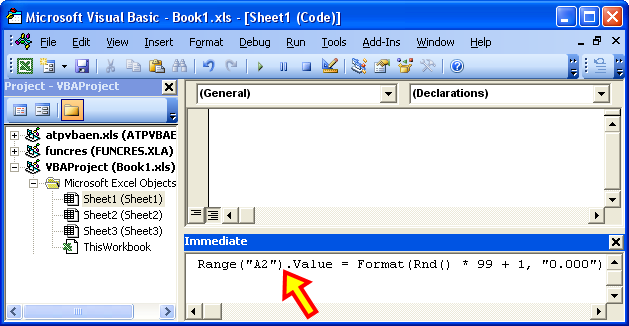From there, same as with Example 1, press `Enter` to place that random number into cell A2, and then press `Alt+Q` to return to your worksheet. Here’s the final result for Example 2: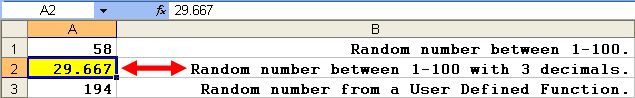Example 3 — the number in cell A3.
Rather than going back and forth between your worksheet and the Immediate window, and further to place a static random number in any cell, you can employ a special kind of formula, called a User Defined Function (UDF), to do the job. There are a couple of one-time steps to take, and then you are home free to use the UDF in that workbook anytime thereafter.
Step 2
In the “Project VBAProject” window, select the bolded name of your workbook. Then, from the menu at the top of the screen, click `Insert > Module`.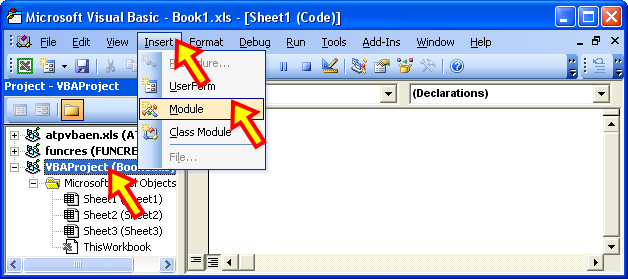Step 3
In the new module created in Step 2, I entered this UDF to return a number between 1 and 365:
`Function StaticRand() As DoubleStaticRand = Int(Rnd() * 365)End Function`
Step 5
Now in any cell, you’d enter your UDF just like this, and as seen in the next picture for cell A3.
`=StaticRand()`
Tom’s Tutorials For Excel: Ranking a List in Random Order
Here’s an example of setting up your worksheet to randomly rank a list of items. In this first set of pictures, the list of names is randomly ranked three times, simply by hitting the `F2` key on any cell in the worksheet, and pressing `Enter`.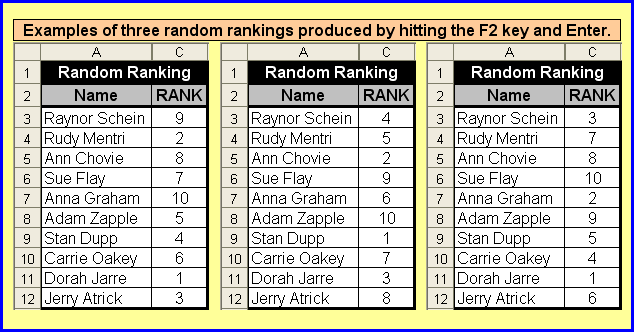In the next pair of side-by-side screen shots, Step 1 is to enter the `RAND()` function into the cells of a helper column. In Step 2, the formula for the ranking column, `=RANK(B3,\$B\$3:\$B\$12)`, is entered into cell C3 and copied down to cell C12.Finally, as seen in this last par of side-by-side screen shots that produce the final result, helper column B is optionally hidden.
Tom’s Tutorials For Excel: Sorting a List in Random Order
Here’s how you can sort a table in a random order, instead of strictly as ascending or descending.
In the example, a company has 10 coveted parking spaces that are nearer to the office building. Because there are more than 10 employees, a weekly lottery is held, using the `RAND` function, to determine which 10 lucky employees get to park in the preferred parking spaces.

Enter the `RAND()` function as a one-time only step to set up your worksheet.
Column B is empty and hidden so as to not be a part of the Sort range.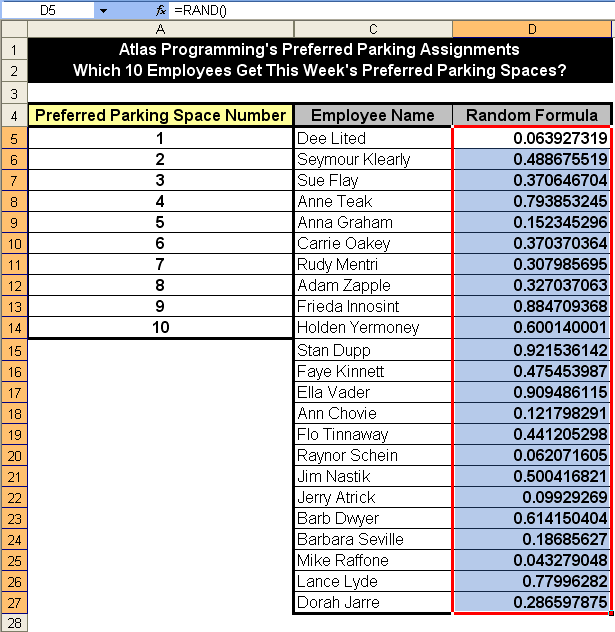Select the range to be sorted. In the example pictured below, it is range C4:D27.
From your keyboard, press `Alt+D+S` to show the Sort dialog box.If you are using version 2003, in the Sort dialog box:
• You can sort by Ascending or Descending; I selected Ascending.
• Be sure to indicate that your sort range has a header row.
• Click OK.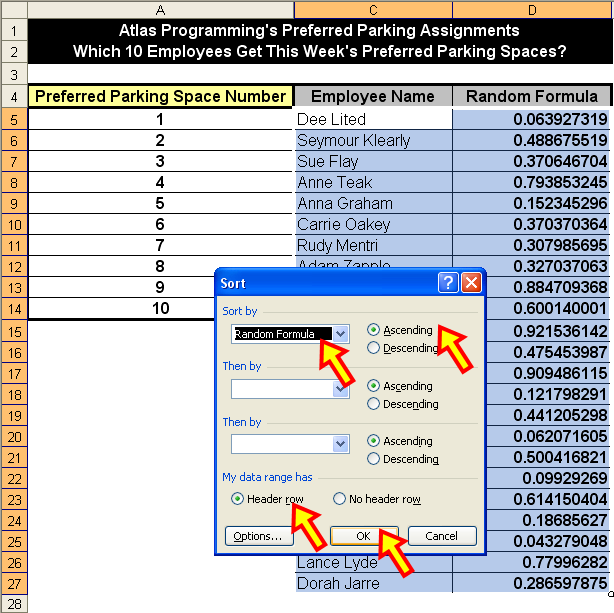If you are using version 2007 or after, in the Sort dialog box:
• Select (put a checkmark in the box nest to) “My data has headers”.
• Sort on Values.
• Sort by Smallest to Largest, or by Largest to Smallest (ascending or descending).
• Click OK.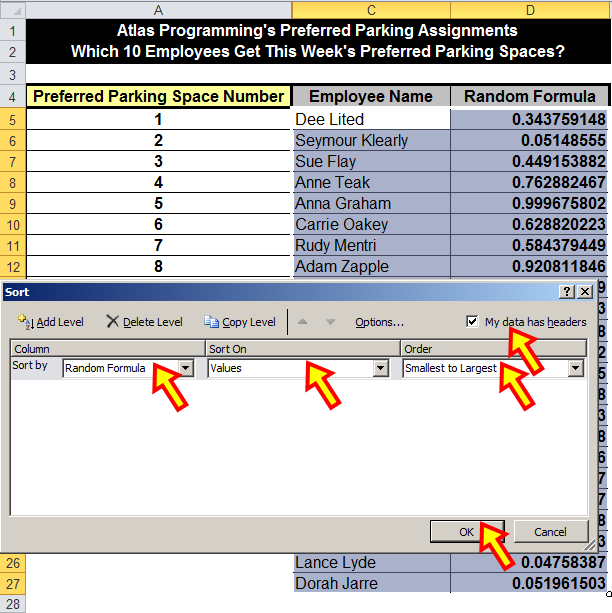Tom’s Tutorials For Excel: Using the RAND Function to Extract Random Data
Here are examples of how numbers or data can be extracted using the `RAND` function.
The examples and formulas listed below relate to the following picture.
Example 1: Randomly return a number between numbers, such as from 1 to 10.
Formula in cell A4 is `=INT(RAND()*10)+1`
Example 2: Example 1 in practice, to randomly return an item in a 10-item list.
Formula in cell E7 is `=INDEX(A8:A17,INT(RAND()*(10)+1))`
Example 3: Similar to Example 1, this time from 65 to 90.
Formula in cell A20 is `=INT(RAND()*(91-65)+65)`
Example 4: Example 3 in practice, to randomly return a letter of the alphabet.
Formula in cell A23 is `=CHAR(INT(RAND()*(91-65)+65))`
Example 5: Random time between two times such as from 9:00 AM and 2:00 PM.
Formula in cell A26 is `=RAND()*(TIME(9,0,0)-TIME(14,0,0))+TIME(14,0,0)`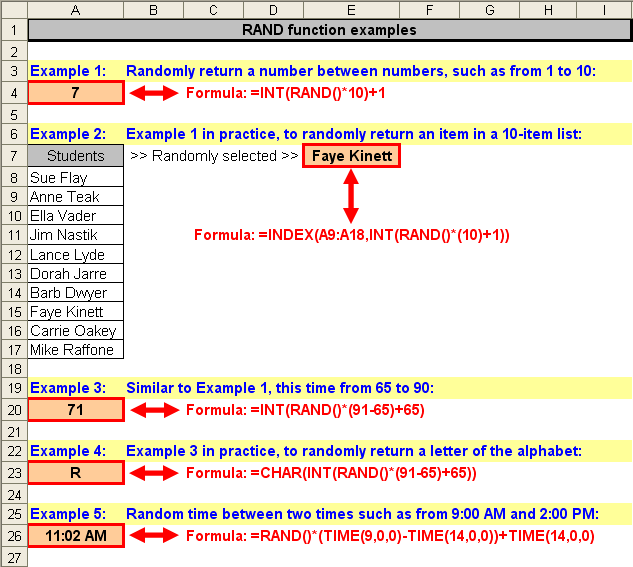📤You download App EVBA.info installed directly on the latest phone here : https://www.evba.info/p/app-evbainfo-setting-for-your-phone.html?m=1